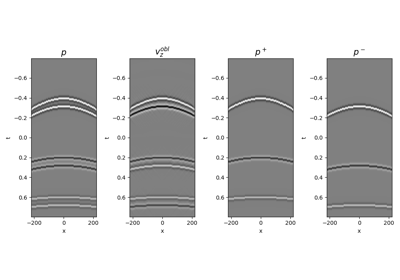# pylops.waveeqprocessing.UpDownComposition2D¶

pylops.waveeqprocessing.UpDownComposition2D(nt, nr, dt, dr, rho, vel, nffts=(None, None), critical=100.0, ntaper=10, scaling=1.0, dtype='complex128')[source]

2D Up-down wavefield composition.

Apply multi-component seismic wavefield composition from its up- and down-going constituents. This input model required by the operator should be created by flattening the concatenated separated wavefields of size $$\lbrack n_r \times n_t \rbrack$$ along the spatial axis.

Similarly, the data is also a concatenation of flattened pressure and vertical particle velocity wavefields.

Parameters: nt : int Number of samples along the time axis nr : int Number of samples along the receiver axis dt : float Sampling along the time axis dr : float Sampling along the receiver array rho : float Density along the receiver array (must be constant) vel : float Velocity along the receiver array (must be constant) nffts : tuple, optional Number of samples along the wavenumber and frequency axes critical : float, optional Percentage of angles to retain in obliquity factor. For example, if critical=100 only angles below the critical angle :mathfrac{f(k_x)}{vel} will be retained ntaper : float, optional Number of samples of taper applied to obliquity factor around critical angle scaling : float, optional Scaling to apply to the operator (see Notes for more details) dtype : str, optional Type of elements in input array. UDop : pylops.LinearOperator Up-down wavefield composition operator

WavefieldDecomposition
Wavefield decomposition

Notes

Multi-component seismic data ($$p(x, t)$$ and $$v_z(x, t)$$) can be synthesized in the frequency-wavenumber domain as the superposition of the up- and downgoing constituents of the pressure wavefield ($$p^-(x, t)$$ and $$p^+(x, t)$$) as follows :

$\begin{split}\begin{bmatrix} \mathbf{p}(k_x, \omega) \\ \mathbf{v_z}(k_x, \omega) \end{bmatrix} = \begin{bmatrix} 1 & 1 \\ \frac{k_z}{\omega \rho} & - \frac{k_z}{\omega \rho} \\ \end{bmatrix} \begin{bmatrix} \mathbf{p^+}(k_x, \omega) \\ \mathbf{p^-}(k_x, \omega) \end{bmatrix}\end{split}$

which we can write in a compact matrix-vector notation as:

$\begin{split}\begin{bmatrix} \mathbf{p} \\ s*\mathbf{v_z} \end{bmatrix} = \begin{bmatrix} \mathbf{F} & 0 \\ 0 & s*\mathbf{F} \end{bmatrix} \mathbf{W} \begin{bmatrix} \mathbf{F}^H & 0 \\ 0 & \mathbf{F}^H \end{bmatrix} \mathbf{p^{\pm}}\end{split}$

where $$\mathbf{F}$$ is the 2-dimensional FFT (pylops.signalprocessing.FFT2), $$\mathbf{W}$$ is a weighting matrix implemented via pylops.basicprocessing.Diagonal, and $$s$$ is a scaling factor that is applied to both the particle velocity data and to the operator has shown above. Such a scaling is required to balance out the different dynamic range of pressure and particle velocity when solving the wavefield separation problem as an inverse problem.

As the operator is effectively obtained by chaining basic PyLops operators the adjoint is automatically implemented for this operator.

  Wapenaar, K. “Reciprocity properties of one-way propagators”, Geophysics, vol. 63, pp. 1795-1798. 1998.

## Examples using pylops.waveeqprocessing.UpDownComposition2D¶12. Seismic wavefield decomposition